Courses

# Friction MCQ Level - 1

## 20 Questions MCQ Test | Friction MCQ Level - 1

Description
This mock test of Friction MCQ Level - 1 for Class 11 helps you for every Class 11 entrance exam. This contains 20 Multiple Choice Questions for Class 11 Friction MCQ Level - 1 (mcq) to study with solutions a complete question bank. The solved questions answers in this Friction MCQ Level - 1 quiz give you a good mix of easy questions and tough questions. Class 11 students definitely take this Friction MCQ Level - 1 exercise for a better result in the exam. You can find other Friction MCQ Level - 1 extra questions, long questions & short questions for Class 11 on EduRev as well by searching above.
QUESTION: 1

### A passenger is travelling a train moving at 40 ms-1.His suitcase is kept on the berth. The driver of trainapplies breaks such that the speed of the traindecreases at a constant rate to 20 ms-1 in 5 s. Whatshould be the minimum coefficient of friction betweenthe suitcase and the berth if the suitcase is not toslide during retardation of the train?

Solution:

Solution :- Retardation of train =204 = 5ms2

it act in the backward direction friction force on suitcase 5m newton , where m is the mass of suitcase in acts in the forward direction due to this force the suitcase has a tendency to slide forward if suitcase is not to slide then 5m = force f of friction

or 5m = mmg or m = 5/10

= 0.5

QUESTION: 2

Solution:
QUESTION: 3

### A block of mass m, lying on a horizontal plane, isacted upon by a horizontal force P and another forceQ, inclined at an angle q to the vertical. The block willremain in equilibrium if the coefficient of frictionbetween it and the surface is (assume P> Q)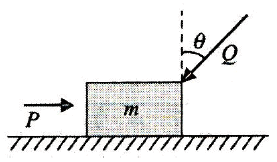Solution:
QUESTION: 4

A horizontal force of 25 N is necessary to just hold ablock stationary against a wall. The coefficient offriction between the block and the wall is 0.4. Theweight of the block is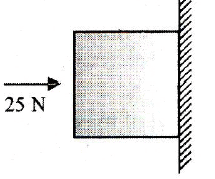Solution:
QUESTION: 5

25A solid block of mass 2 kg is resting inside a cubeas shown in the figure. The cube is moving with avelocity v = 5iˆ + 2 ˆjms-1 r .If the coefficient of frictionbetween the surface of cube and block is 0.2, thenthe force of friction between the block and cube is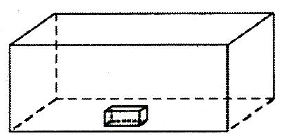Solution:
QUESTION: 6

27In the given figure, the blocks are at rest and a forceof 10 N acts on the block of 4 kg mass. The cofficientof static friction and the coefficient of kinetic frictionare μs = 0.2 and μk = 0.15 for both the surfaces incontact. The magnitude of friction force actingbetween the surface of contact between the 2 kgand 4 kg block in this situation is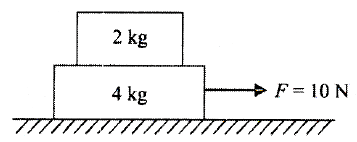Solution:
QUESTION: 7

28The masses of the blocks A and B are m and M,respectively. Between A and B there is a constantfrictional force F, and B can slide frictionlessly onhorizontal surface. A is set in motion with velocitywhile B is at rest. What is the distance moved by Arelative to B before they move with the same velocity?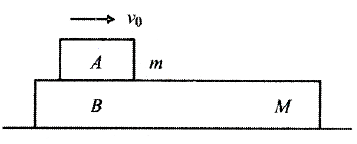Solution:
QUESTION: 8

Three blocks A,B and C of equal mass m are placedone over the other on a frictionless surface (table)as shown in the figure. Coefficient of friction betweenany blocks A,B and C is μ. The maximum value ofmass of block D so that the blocks A,B and C movewithout slipping over each other is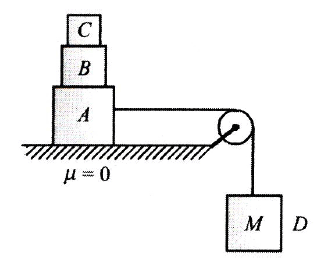Solution:
QUESTION: 9

Two blocks of masses 0.2 kg and 0.5 kg, which areplaced 22 m apart on a rough horizontal surface(μ = 0.5), are acted upon by two forces of magnitude3 N each as shown in figure at time t = 0. Then, thetime t at which they collide with each other is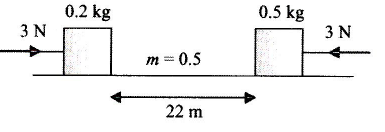Solution:
QUESTION: 10

A block of mass 2 kg is placed on the floor. Thecoefficient of static friction is 0.4. If a force of 2.8 N isapplied on the block parallel to floor, the force of frictionbetween the block and floor is : (Taking g = 10 m/s2)

Solution:
QUESTION: 11

A body of mass 2 kg rests on a rough inclined planemaking an angle 30° with the horizontal. The coefficientof static friction between the block and the plane is0.7. The frictional force on the block is:

Solution:
QUESTION: 12

A block has been placed on an inclined plane. Theslope angle q of the plane is such that the block slidesdown the plane at a constant speed. The coefficient ofkinetic friction is equal to:

Solution:
QUESTION: 13

A body of mass 60 kg is dragged with just enoughforce to start moving on a rough surface with coefficientsof static and kinetic frictions 0.5 and 0.4 respectively.On applying the same force, what is the acceleration?

Solution:
QUESTION: 14

A block A of mass 2 kg rests on another block B ofmass 8 kg which rests on a horizontal floor. Thecoefficient of friction between A and B is 0.2 while thatbetween B and floor is 0.5. When a horizontal force of25 N is applied on the block B, the force of frictionbetween A and B is :

Solution:
QUESTION: 15

A 40 kg slab rests on a frictionless floor. A 10 kg blockrests on top of the slab (Fig). The static coefficient offriction between the block and the slab is 0.60 whilethe kinetic coefficient is 0.40. The 10 kg block is actedupon by a horizontal force of 100 N. If g =9.8 m/s2 theresulting acceleration of the slab will be: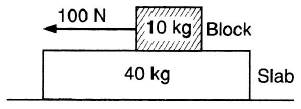Solution:
QUESTION: 16

A mass m rests on a horizontal surface. The coefficientof friction between the mass and the surface is μ. If themass is pulled by a force F as shown in Fig., the limitingfriction between the mass and the surface will be: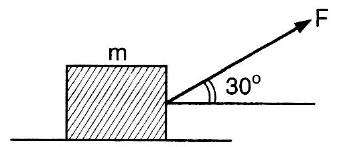Solution:
QUESTION: 17

A block of mass 0.1 kg is held against a wall by applyinga horizontal force of 5 N on the block. If the coefficientof friction between the block and the wall is 0.5, themagnitude of the frictional force acting on the block is:

Solution:
QUESTION: 18

A lineman of mass 60 kg is holding a vertical pole. Thecoefficient of static friction between his hands and thepole is 0.5. If he is able to climb up the pole, what isthe minimum force with which he should press the polewith his hands? (g = 10 m/s2)

Solution:
QUESTION: 19

A box weighing 20 kg is pushed along the floor at aconstant speed by applying a horizontal force. If thecoefficient of friction is 0.25, then force applied is :

Solution:
QUESTION: 20

A block takes twice as much time to slide down a 450rough inclined plane as it takes to slide down a similarsmooth plane. The coefficient of friction is :

Solution: